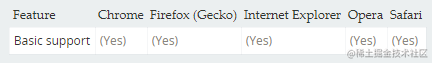如何解决0.1 +0.2===0.30000000000000004类问题一.类库部分

math.js

math.js是JavaScript和Node.js的一个广泛的数学库。支持数字，大数，复数，分数，单位和矩阵等数据类型的运算。

GitHub：github.com/josdejong/m…

0.1+0.2 ===0.3实现代码：

var math = require('mathjs')

decimal.js

GitHub：github.com/MikeMcl/dec…

var Decimal = require('decimal.js')
x = new  Decimal(0.1)
y = 0.2
console.log(x.plus(y).toString())//'0.3'复制代码

bignumber.js

Github：github.com/MikeMcl/big…

var BigNumber = require("bignumber.js")
x = new BigNumber(0.1)
y = 0.2
console.log(x.plus(y).toString())//'0.3'复制代码

big.js

Github：github.com/MikeMcl/big…

var Big = require("big.js")
x = new Big(0.1)
y = 0.2
console.log(x.plus(y).toString())//'0.3'复制代码

math.js：npm.runkit.com/mathjs
big.js： npm.runkit.com/big.js

二、原生方法

toFixed() 方法

toFixed() 方法使用定点表示法来格式化一个数，会对结果进行四舍五入。语法为：

JavaScript 代码:
numObj.toFixed(digits)

JavaScript 代码:

parseFloat((数学表达式).toFixed(digits))； // toFixed() 精度参数须在 0 与20 之间
// 运行
parseFloat((0.1 + 0.2).toFixed(10))//结果为0.3
parseFloat((0.3 / 0.1).toFixed(10)) // 结果为 3
parseFloat((0.7 * 180).toFixed(10))//结果为126
parseFloat((1.0 - 0.9).toFixed(10)) // 结果为 0.1
parseFloat((9.7 * 100).toFixed(10)) // 结果为 970
parseFloat((2.22 + 0.1).toFixed(10)) // 结果为 2.32复制代码

desktop端：mobile端：